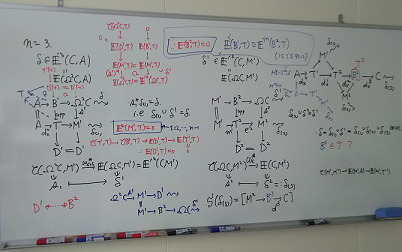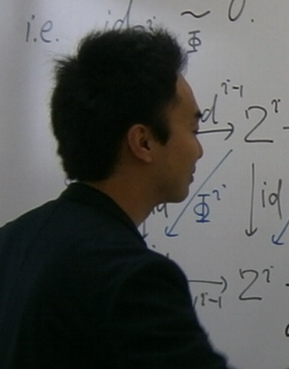Mathematics and Computer Science

Associate professor

Nakaoka, Hiroyuki

# Categorical frameworks appearing in algebra

## Generalization and abstraction in algebra

Sometimes, generalization and abstraction play an important role in mathematics. For example, as the set of integers and the set of polynomials (e.g. with real coefficients) have many similar algebraic properties, we may expect to deal with them in a common framework. Indeed, by abstracting the structures of addition and multiplication with appropriate conditions, we obtain the notion of rings, which contains them as examples. Study of rings gives us a general theory which is applicable to these examples.
Or, let us remember the undergraduate courses of linear algebra and group theory. In linear algebra we deal with vector spaces, which are sets equipped with additions and scalar multiplications. In group theory, groups are sets equipped with appropriate multiplications. Also in these cases, by extracting structures possessed by examples, we deduce general properties with wide range of applications.
On the other hand, it is also natural that these abstract notions themselves are of interests as mathematical subjects, along with the development of such general theories. Then, as a further abstraction, one may ask about a framework to unify them. Although it is just one aspect, category theory enables us to realize this by means of arrows.

## Categories as frameworks

In the long history of mathematics, category theory is a relatively new field, born about 70 years ago. Because of its high level of abstraction, category theory can be applied widely, and enables us to describe various things very roughly in terms of arrows. Properties of vector spaces, groups, and rings are investigated by using linear maps and homomorphisms, which can be regarded as arrows in categories. In fact, categories exist not only in algebra, but are present in many places where mathematical objects and arrows are considered. Recently, they also appear outside of mathematics.
The objective of my research is to catch the frameworks found in algebra, using category theory. It may be a bit special, in the sense that I am rather interested in tools rather than naïve mathematical objects. Mainly I am studying categorical notions appearing around finite group theory and the representation theory of rings. Especially, I usually engage in homological algebra. The most important classes of categories in homological algebra are abelian categories and triangulated categories, both of which deeply involve the works of Alexander Grothendieck. These days, I am working on a unification of these two classes of categories.#### Profile

Mathematics and Computer Science

Associate professor

Nakaoka, Hiroyuki

Though it varies slightly depending on the academic year, I am teaching the undergraduate courses on group theory (Algebra I) and ring theory (Algebra II). In the graduate school, I usually give a course on category theory as Advanced Algebra. I am specializing in category theory, studying categories around finite group theory and the representation theory of rings. If possible, I would like to start research on categories and related notions in other areas of mathematics in the near future.

### Message for Students

Amongst all requirements in doing mathematics, such as solid understanding and accurate arguments, one of the most important for continuing research is <<interest>>. As the world of mathematics is widely ranged in many different directions, the way people get interested in mathematics can also be diverse. I hope you will find your interest in mathematics deepen, through your study of mathematics.